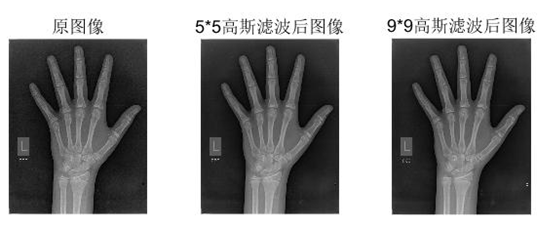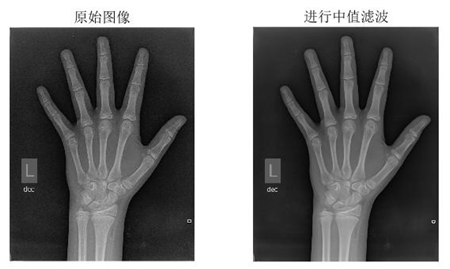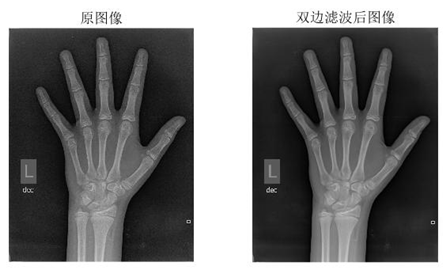• ## Matlab中值滤波代码

千次阅读 2016-10-30 19:38:58
%中值滤波,接受两个参数，一个参数是原图像x,另一个参数是滤波器大小n function d=median_filter(x,n) d=x; [width,height]=size(x);%得到图像的长和宽 for ii=1:width-(n-1)  for jj=1:height-(n-1)%...
img=imread('lena.png');img=rgb2gray(img)img=im2double(img)%输入图像类型为uint8,将其转换为double类型进行运算gau=imnoise(img,'gaussian');subplot(2,2,1);imshow(gau);title('添加高斯噪声的图像');%imshow()函数在显示图像时会自动将类型转换为uint8subplot(2,2,2);imshow(median_filter(gau,3));title('滤除高斯噪声后的图像');salt=imnoise(img,'salt & pepper');subplot(2,2,3);imshow(salt);title('添加椒盐噪声的图像');subplot(2,2,4);imshow(median_filter(salt,3));title('滤除椒盐噪声后的图像');

%中值滤波,接受两个参数，一个参数是原图像x,另一个参数是滤波器大小n
function d=median_filter(x,n)
d=x;
[width,height]=size(x);%得到图像的长和宽
for ii=1:width-(n-1)
for jj=1:height-(n-1)%height表示的个数为可完整滤波的格子数
tmp1=d(ii:ii+(n-1),jj:jj+(n-1));%取出要滤波的n*n的方阵
tmp2=tmp1(1,:);
for kk=2:n
tmp2=[tmp2,tmp1(kk,:)];%把所有的行排成一行方便后面求中值
end
y= median(tmp2);
d(ii+(n-1)/2,jj+(n-1)/2)=y;
end
end


展开全文• matlab中值滤波及邻域滤波对椒盐噪声，高斯噪声的不同模板处理
• matlab中值滤波邻域平滑高斯滤波图像处理结果比较程序代码
• 中值过滤代码matlab 中值过滤器 用于MATLAB的快速中值滤波器 用于MATLAB中深度帧中值滤波的C ++兼容代码。 RGBD相机会收集一个深度框，该深度框可能包含缺少的像素值。
• 基于变异的中值滤波取证 论文的作者仅出于研究目的（此方法与其他方法的评估和比较）提供了此MATLAB代码。 参考文献 Kang Hyeon RHEE，“使用相邻线对的变化进行中值滤波检测以进行图像取证”，电子成像杂志，...
• 中值滤波代码matlab Application_Perfections_Citra GUI应用程序，使用中值滤波方法校正图像中的噪声 Nama : Arif Samsudin NIM : 311910255 Kelas : TI 19 C1 1.准备创建GUI应用程序 在命令窗口中输入指南，然后按...
• 中值滤波代码 matlab simple_image_process Matlab code for means filter and median filter
• 中值滤波代码 matlab Modified-Deciision-based-median-filter Matlab Code
• 中值滤波代码 matlab improved-median-filter More details plz visit This program outcome better than inbuild medfilt2 function
• matlab代码中值滤波，比较简单，适合初学者使用，直接运行即可。
• 自己编写的中值滤波matlab程序，主要是对图像去噪声
• 中值滤波代码 matlab median-filter Salt and pepper noise and median filter MATLAB code with and without inbuilt function
• 中值滤波代码matlab 概括 在这个项目中，已经研究了图像处理算法。 先前已从所有算法的范围中选择和准备的报告已被重新阐述，并试图加以准备。 已经研究了九种不同的程序。 从建立要使用的开发环境开始，举例说明...
• matlab图像滤波 1.高斯滤波 I = imread(img); %input the path of image; J1= fspecial('gaussian',[5,5],1); J2= fspecial('gaussian',[9,9],1); I1 = imfilter(I,J1,'replicate'); I2 = imfilter(I,J2,'replicate...
matlab图像滤波
1.高斯滤波
I = imread(img);  %input the path of image;
J1= fspecial('gaussian',[5,5],1);
J2= fspecial('gaussian',[9,9],1);
I1 = imfilter(I,J1,'replicate');
I2 = imfilter(I,J2,'replicate');
subplot(1,3,1),imshow(I),title('原图像');
subplot(1,3,2),imshow(I1);title('5*5高斯滤波后图像');
subplot(1,3,3),imshow(I2);title('9*9高斯滤波后图像');2.中值滤波
I=imread(image);    %input the path of image;
subplot(1,2,1);imshow(I);title('原始图像');
K=medfilt2(I,[9,9]);%进行中值滤波;
subplot(1,2,2);imshow(K);title('进行中值滤波');3.双边滤波
img=imread(image);    %input the path of image;
img=mat2gray(img);
[m,n]=size(img);
subplot(121);imshow(img);title('原图像');
r=10;        %模板半径
imgn=zeros(m+2*r+1,n+2*r+1);
imgn(r+1:m+r,r+1:n+r)=img;
imgn(1:r,r+1:n+r)=img(1:r,1:n);                 %扩展上边界
imgn(1:m+r,n+r+1:n+2*r+1)=imgn(1:m+r,n:n+r);    %扩展右边界
imgn(m+r+1:m+2*r+1,r+1:n+2*r+1)=imgn(m:m+r,r+1:n+2*r+1);    %扩展下边界
imgn(1:m+2*r+1,1:r)=imgn(1:m+2*r+1,r+1:2*r);       %扩展左边界
sigma_d=2;
sigma_r=0.1;
[x,y] = meshgrid(-r:r,-r:r);
w1=exp(-(x.^2+y.^2)/(2*sigma_d^2));     %以距离作为自变量高斯滤波器
h=waitbar(0,'wait...');
for i=r+1:m+r
for j=r+1:n+r
w2=exp(-(imgn(i-r:i+r,j-r:j+r)-imgn(i,j)).^2/(2*sigma_r^2)); %以周围和当前像素灰度差值作为自变量的高斯滤波器
w=w1.*w2;

s=imgn(i-r:i+r,j-r:j+r).*w;
imgn(i,j)=sum(sum(s))/sum(sum(w));
end
waitbar(i/m);
end
close(h)
subplot(122);imshow(mat2gray(imgn(r+1:m+r,r+1:n+r)));title('双边滤波后图像');展开全文• 中值过滤代码matlab Matlab中的自适应图像滤镜 描述 您会发现以下自适应过滤器： 双边过滤器 桑原过滤器 中值增长过滤器 三态中值滤波器 均方误差滤波器 软件需求 致谢 特别感谢谁帮助制定了代码
• matlab影像中值滤波去噪声代码图像过滤 MATLAB中的降噪实验。 1）ADID NOISE（油性噪声）Inoisy = imnoise（I，'salt＆pepper'，d）％脉冲噪声 2）对于脉冲噪声，“ d”是受影响像素的比例。 保持为0.05、0.1和0.2。...
• 中值过滤代码matlab 社交网站 稳定浅水波代码仓库 ...中值滤波替代 展开阶段，使用样条曲线？ （或者是其他东西） 长期〜1个月 二维码 数据管理+存储 易于使用-制作一个“如何运行代码”文档 自动图像裁剪
• 利用simulink搭建仿真模型对自适应中值滤波算法进行验证，结果表明所设计系统的有效性和自适应中值滤波算法的准确性simulink
• 现在我们必须使用均值滤波和中值滤波对噪声图像进行去噪。 我们必须根据噪声类型应用适当的滤波器。 要计算高斯蒙版的权重，请使用 5）还附有用于PSNR的代码-用于计算PSNR值。 6）原始（干净）图像和去噪后的图像...
• 最近数字图像处理课程课后作业，要求自己用代码实现自适应中值滤波器，虽然很简单，但是在网上找了一圈也没有可以直接拿来用的，所以就在网上找了一段代码，并且自己改了一下，能够实现效果了，虽然效率不是很高，...
最近数字图像处理课程课后作业，要求自己用代码实现自适应中值滤波器，虽然很简单，但是在网上找了一圈也没有可以直接拿来用的，所以就在网上找了一段代码，并且自己改了一下，能够实现效果了，虽然效率不是很高，但是能用，希望有需要的人也可以拿去用

代码

一共三段代码，主函数以及两个方法

主函数
img_rgb = imread('D:\1.jpg');
img_gray = rgb2gray(img_rgb);
imshow(img_gray);
title('原图');
figure();
imshow(img_gray);
img_salt = imnoise(img_gray,'salt & pepper',0.4);
imshow(img_salt);
title('加入0.4椒盐噪声');
figure();
img_gaussian = imnoise(img_gray,'gaussian',0.3);
imshow(img_gaussian);
title('加入0.3高斯噪声');
figure();
imshow(medfilt2(img_salt),[3,3]);
title('中值滤波处理椒盐噪声图像');
figure();
imshow(medfilt2(img_gaussian,[3,3]));
title('中值滤波处理高斯噪声图像');
figure();
imshow(RAMF(img_salt,3));
title('椒盐噪声图像自适应中值滤波后图片');
figure();
imshow(RAMF(img_gaussian,4));
title('高斯噪声图像自适应中值滤波后图片');

自适应中值滤波方法
function img_RAMF = RAMF(img_noise, max)

%获取图像的列数
m=size(img_noise,1);
%获取图像的列数
n=size(img_noise,2);

%确定最大的滤波半径
Nmax=max;

%下面是边界扩展，图像上下左右各增加Nmax像素。
imgn=zeros(m+2*Nmax,n+2*Nmax,'uint8');
imgn(Nmax+1:m+Nmax,Nmax+1:n+Nmax)=img_noise;

imgn(1:Nmax,Nmax+1:n+Nmax)=img_noise(1:Nmax,1:n);                 %扩展上边界
imgn(1:m+Nmax,n+Nmax+1:n+2*Nmax+1)=imgn(1:m+Nmax,n:n+Nmax);    %扩展右边界
imgn(m+Nmax+1:m+2*Nmax+1,Nmax+1:n+2*Nmax+1)=imgn(m:m+Nmax,Nmax+1:n+2*Nmax+1);    %扩展下边界
imgn(1:m+2*Nmax+1,1:Nmax)=imgn(1:m+2*Nmax+1,Nmax+1:2*Nmax);       %扩展左边界

re=imgn;
for i=Nmax+1:m+Nmax
for j=Nmax+1:n+Nmax

r=1;                %初始滤波半径
while r <= Nmax
W=imgn(i-r:i+r,j-r:j+r);
W = my_sort(W);
W_size = size(W);
Imin=W(1);
Imax=W(W_size(1));
Imed=W(ceil(W_size(1)/2));
if Imin<Imed && Imed<Imax       %如果当前邻域中值不是噪声点，那么就用此次的邻域
break;
else
r=r+1;              %否则扩大窗口，继续判断
end
end

if Imin<imgn(i,j) && imgn(i,j)<Imax         %如果当前这个像素不是噪声，原值输出
re(i,j)=imgn(i,j);
else                                        %否则输出邻域中值
re(i,j)=Imed;
end

end
end

img_RAMF = re(Nmax+1:m+Nmax,Nmax+1:n+Nmax);

排序方法
function sort_data = my_sort(data)

data_size = size(data);
width = data_size(2);
height = data_size(1);
sort_data = data(:);

data_length = width * height;
for i = 1:data_length - 1
for j = i + 1:data_length -1
if sort_data(i) > sort_data(j)
x = sort_data(i);
sort_data(i) = sort_data(j);
sort_data(j) = x;
end
end
end


展开全文• 自己写的基于MATLAB的去除图像高斯噪声的中值滤波代码，已经仿真过。希望可以帮到大家
• 中值过滤代码matlab 快速发现 简单，快速的2D峰值查找器。 目的是要比更复杂的技术更快，但又足以在嘈杂的数据中找到峰值。 该代码分析嘈杂的2D图像，并使用鲁棒的局部最大值查找器（1像素分辨率）或加权质心（子...
• MATLAB源程序代码分享：MATLAB实现图像的中值滤波 均值滤波 维纳滤波...

# matlab中值滤波代码matlab 订阅